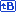# <!-- google_ad_client = "ca-pub-3492315900433860"; /* バナー（468×60） */ google_ad_slot = "9079224868"; google_ad_width = 468; google_ad_height = 60; //--> 数値計算ScilabTop / Scilabhttp://www.scilab.org/

The Free Software for Numerical Computation

## 機能

### Main Features

• Hundreds of mathematical functions
• High level programming language
• 2-D and 3-D graphics
• Advanced data structures and user defined data types
• Xcos: hybrid dynamic systems modeler and simulator

### 2-D and 3-D visualization

• Lines
• Pie charts
• Histograms
• Surfaces
• Animations
• Graphics export in many formats: GIF, BMP, JPEG, SVG, PDF...
• LaTeX / MathML annotations

### Numerical computation

• Linear algebra (線形代数)
• Sparse matrices (疎行列?)
• Polynomials and rational functions
• Simulation: explicit and implicit systems of differential equations solvers
• Classic and robust control
• Differentiable and non-differentiable optimization

### Data analysis

• Interpolation, approximation
• Signal Processing
• Statistics

### Extended features

• Graphs and Networks
• Interface with [Fortran, C, C++, Java
• Functions for calling Scilab from C, C++, Fortran and Java
• LabVIEW to Scilab Gateway
• A large number of external modules available via ATOMS